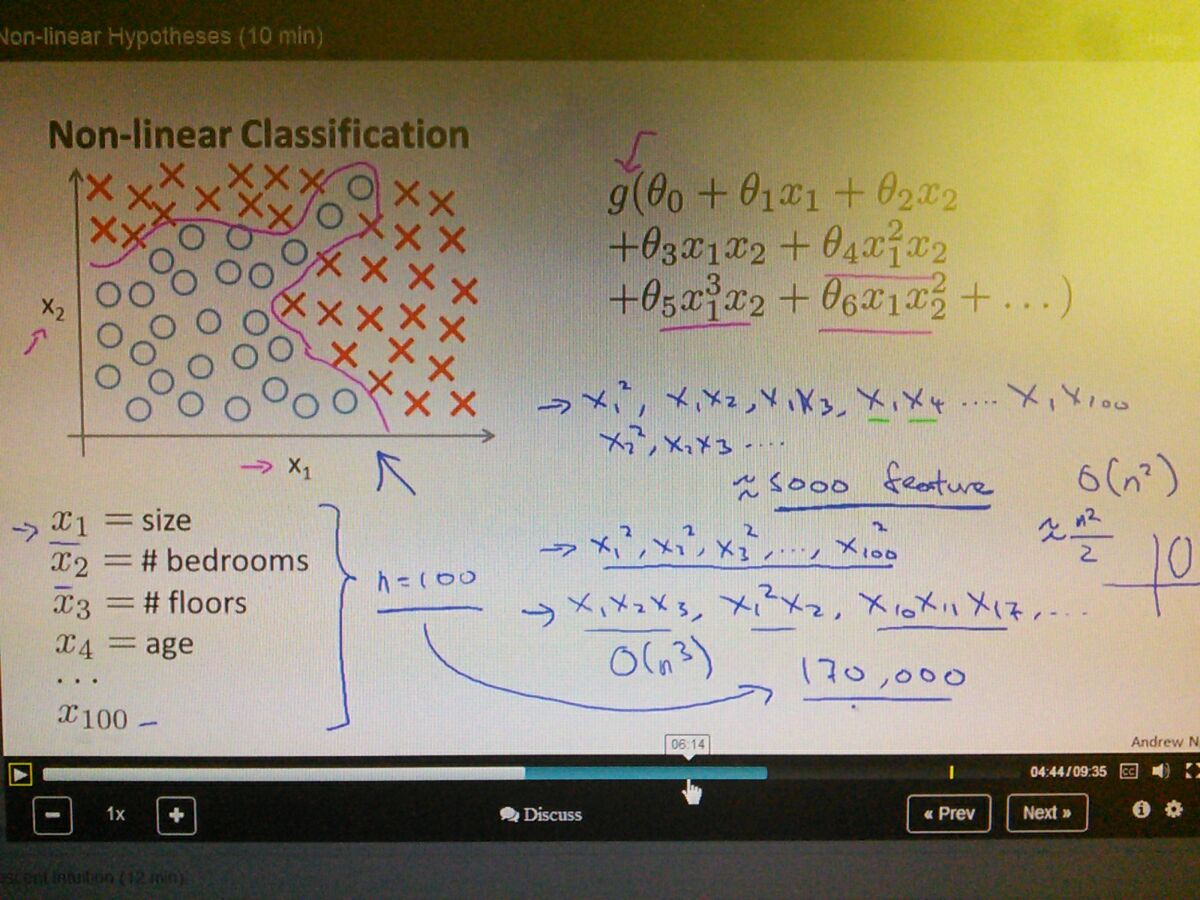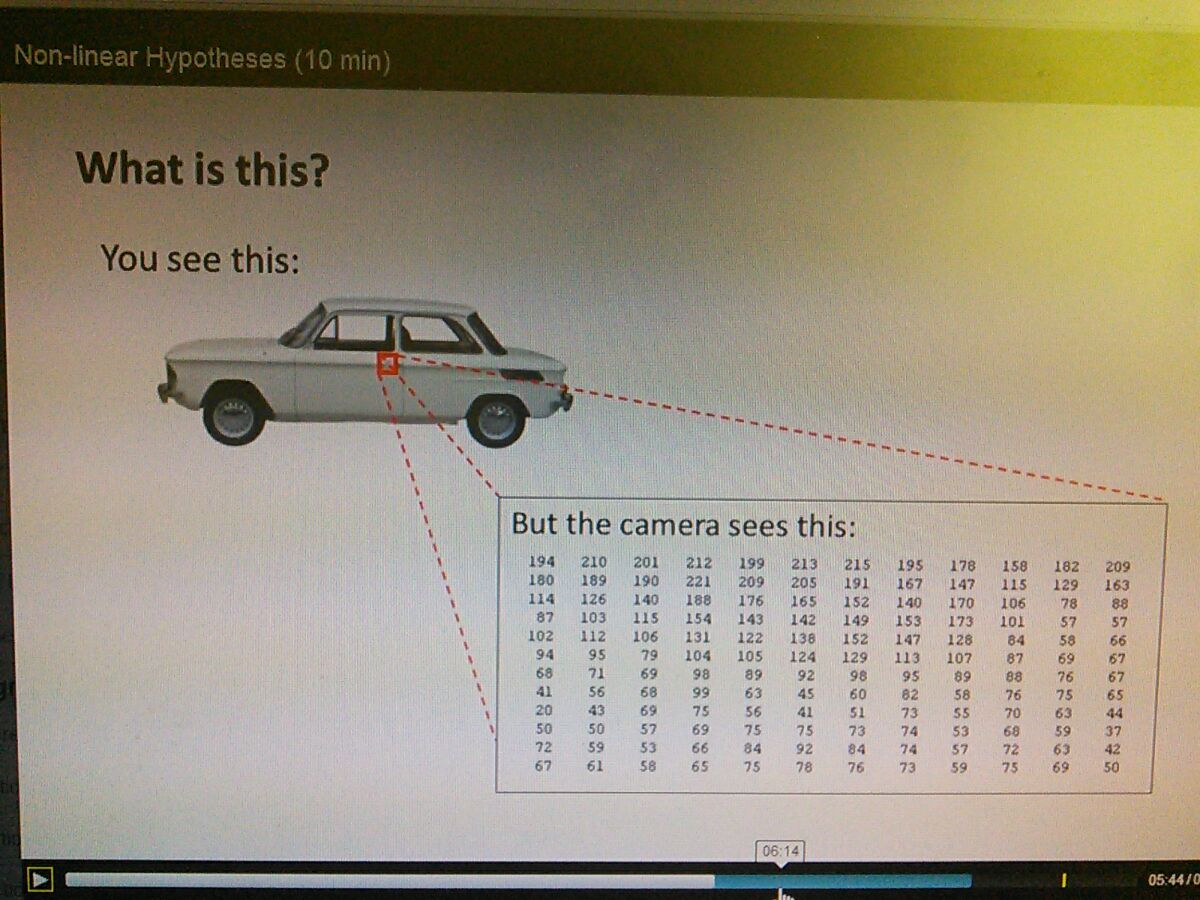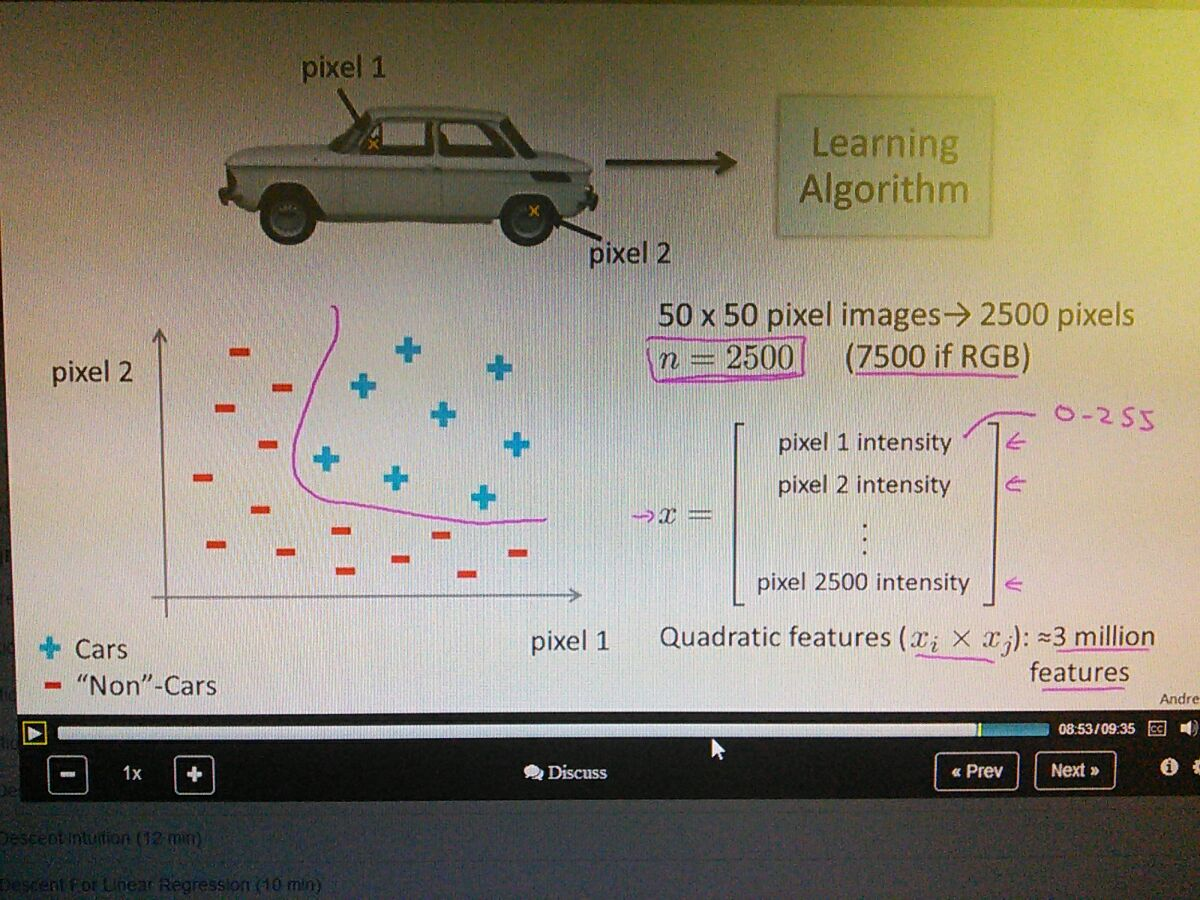• Outdated but most powerful learning algorithm in most ML problems• the number of features is increasing as big theta notation
• even only quadratic feature get picked,  only get circle,  not the more complex one
• if not the the quadratic get picked,  the order will increase a lot more,  close to 170.000 features..
• for many,  examples,  n tend to increase very large• examples of car image
• thr computer vision is hard,  that's way
• if we want to tell computer to recognize the image
• the computer analyze the door knob• need to differentiate the cars and non cars
• high computational expensive
• n = 2500, for grayscale,  7500 for RGB,  3 million if quadratic included
• to put it simply,  the logistic regression is expensive for lots of features
• here's the neural networks actually works,  solving a non-linear problems  with a lot of complex features